# How to use the PMT function in ExcelMicrosoft Excel is a great tool for managing and analyzing all sorts of data. It also has a number of financial functions. This makes it a fantastic resource to perform financial calculations with. One of the main financial functions in Excel is the PMT function. PMT stands for payment, which is what the function is used for: calculate recurring payments over a defined period of time, given the interest rate and present value of the loan.

Essentially, the PMT function allows you to perform time-value of money calculations. To determine the regular payments for a mortgage or line of credit in a very easy format, for example.

### Syntax

=PMT(rate, nper, pv, [fv], [type])
• rate is the interest rate per period, expressed in decimal or percentage format. So if your periods are in years, and your interest rate is 12% per year, the rate is 0.12 or 12%
• nper is the number of periods that the payments are spread across. These periods can be anything: years, months, weeks, days, etc. You just need to make sure that the rate is expressed in the same units as the periods. For example, if your periods are in months, then the 12% yearly interest rate should also be expressed in months (12% per year / 12 months = 1% per month)
• pv is the present value. If you’re talking about a loan or a mortgage, this would be the principal (i.e. the starting value of the loan at the beginning of the first period).
• fv is an optional parameter which describes the future value. By default, this value is zero. This means that the payments reduce the present value (beginning value) down to a future value of zero, by the end of the last period
• type is an optional parameter that specifies when payments will be made. A type of 0 means that payments are made at the end of each period. Whereas type 1 means that payments are made at the beginning of the period. The default value is 0.

### How to use the PMT function in Excel

#### PMT per year

I’ll start with a basic example where I have a \$1000 loan that I need to pay back in one year. There’s only 1 period since I’m repaying the loan all at once, one year from now.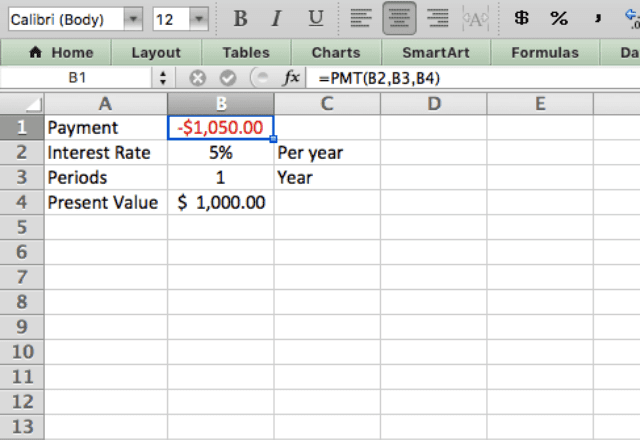I put the following parameters into the function: interest rate (12%), periods (1), and the present value, or principle, of the loan (\$1000). I calculate the payment I’ll need to make by typing =PMT(B2,B3,B4).

As expected, since this was a 1-period loan, the payment is simply the principle (\$1000) plus the interest from the period (5% of \$1000 = \$50).

#### PMT per month

For the next example, I set the period to a monthly basis. This indicates that I’ll be making monthly payments on the same loan instead of one payment at the end of the year. Otherwise, the terms of the loan remain the same as the previous example.

Note that two things change here: the period goes from 1 year to 12 months, and the interest rate goes from 5% to 0.42%. You may wonder why the interest rate changed, when the terms of the loan are the same except for the payment frequency.

The reason for the change is that you have to keep the interest rate and periods in the same units. So when I change from years to months, I have to convert the yearly 5% interest rate into a monthly rate. I do so by dividing it by 12.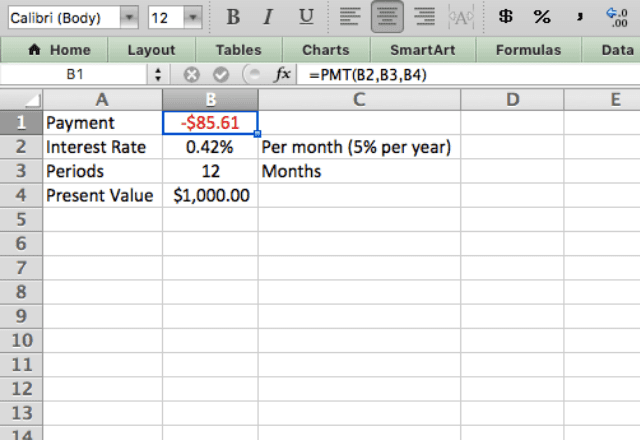Take a closer look at the total amount paid in these first two examples. To calculate the total amount paid, simply multiply the payment value by the number of periods. In the first example I made one payment of \$1050, whilst in the second example my total payments were \$1027.

There’s a difference between yearly and monthly payments because the amount I pay off in the first month (minus interest) reduces the amount of the loan. This means that the interest charged in subsequent months slightly reduces. This happens every month, and the net result is a reduction in the total interest paid over the life of the loan.

#### Mortgage payment

The PMT function in Excel is commonly used for calculating mortgage payments. I do this below for a \$200,000 mortgage with biweekly payments made over the course of 25 years.

The interest rate here is 3% per year, but because the payments are biweekly, you need to divide the 3% by 26 (the number of biweekly payments made per year).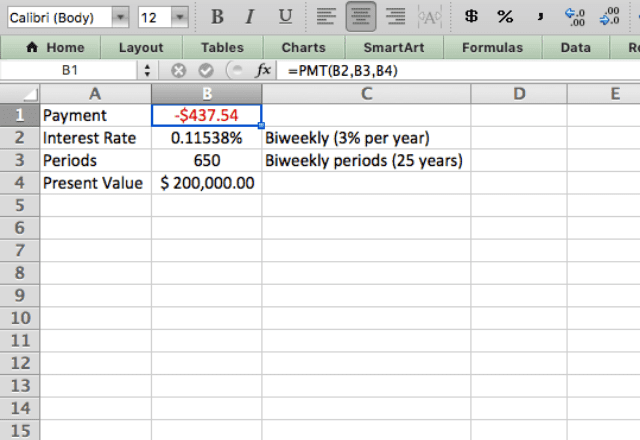You see that the Excel PMT function performs complex calculations such as mortgage payments extremely easily!

#### Type 1 vs. Type 0

You’ve seen a few examples of PMT now, but I haven’t yet incorporated the type parameter in the function. Use this parameter to make payments at the beginning of the period instead of at the end, which is the default (type 0).

I demonstrate the effect of the different payment schedules (start of period vs. end of period) in the screenshot below.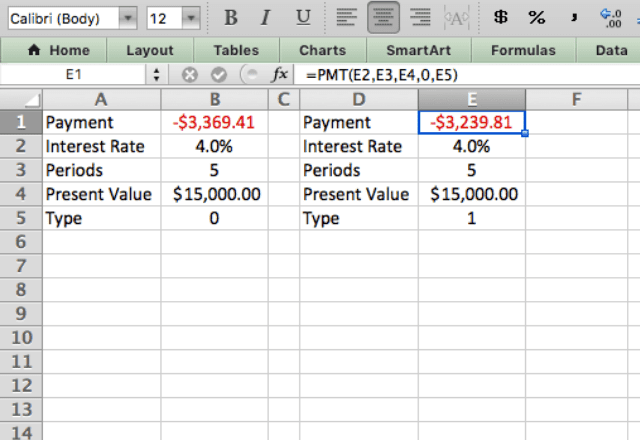Note that there’s a difference in the payment amount between the two types. This is due to the time value of money. You reduce the amount of the loan that accumulates interest during the period by making payments at the beginning of the period (type 1).

This results in an overall reduction in interest, which then reflects in the lower payment.

#### Future value

Now let me demonstrate how the FV (future value) parameter is used.

Remember that by default the future value is 0. So in all the previous examples, I calculated payments that brought the loan down to \$0 by the end of the final period (i.e. I calculated the payments required to pay off the loan completely). Use the FV parameter when this is not the case.

Take a look at the example below. Here, I pretend I’ve been granted a line of credit of \$5000. I will need this money over the next 3 years. I use the PMT function to calculate how much I should take from the line of credit each year, in order to have taken the full amount by the end of year 3.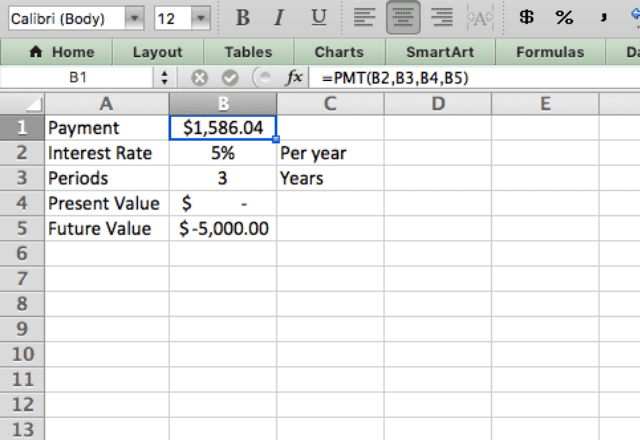Notice that the payments are positive since it’s money I’m getting. And the future value is negative since it’s money that I owe at the end. The result of the function is \$1586.

This means that I should take \$1586 per year in order to maximize my line of credit.

#### Retirement savings

Lastly, I’ll show you how to use the PMT function to calculate monthly retirement savings.

In this situation, pretend that I’m a financial advisor that helps a client plan for retirement. The client wants to retire in 20 years. In order to retire comfortably, she needs to boost her savings by an additional \$180,000.

Assuming an average investment return of 6% per year, how much should the client save each month in order to reach her goals?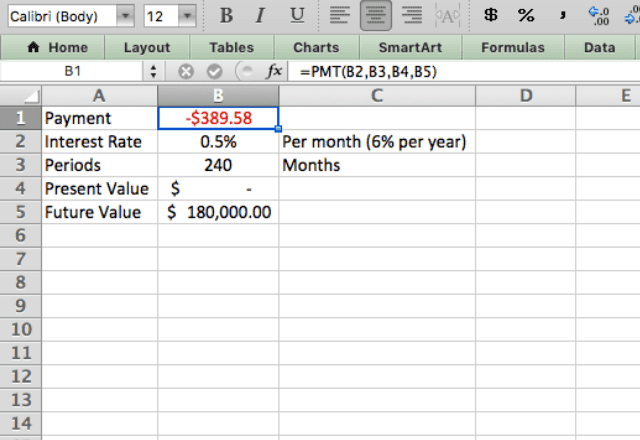I again use the future value parameter in this example. This is because I am concerned about the future value of her retirement savings. I also converted the 6% yearly interest rate into a monthly rate of 0.5%. The number of periods is 240, which is the number of monthly payments the client will make over the course of 20 years.

You might be overwhelmed when you read the description of this retirement plan, but look how simple the calculation really is! I determined that my client needs to save \$389.58 every month for the next 20 years in order to reach her retirement goals.

The Excel PMT function makes all these complicated financial calculations very simple!

### Final tips for the PMT function

• The PMT function always gives equal payments at consistent periods. This means that you can’t use the function if you want to pay different amounts at different times. It’s an ideal function for mortgage calculations, which typically have fixed periodic payments over the term of the mortgage
• Always make sure your periods and interest rate are in the same units (e.g. if the periods are in weeks, the interest rate should be % per week, which you find by dividing the yearly interest rate by 52)
• Notice the signs (negative and positive) of the numbers. Typically, payment values are negative, whereas PV amounts are positive if you are receiving money from a loan
• Calculate the total amount paid by multiplying the payment by the number of periods